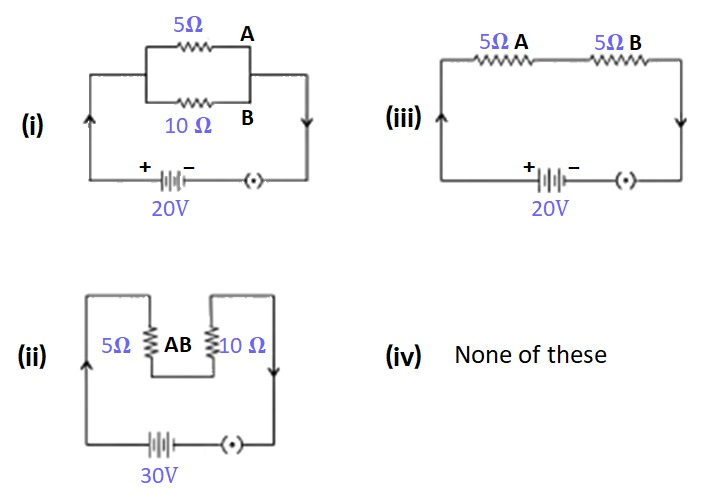Case Based Questions (MCQ)

Class 10
Chapter 12 Class 10 - Electricity

##googletag.cmd.push(function() { googletag.display('div-gpt-ad-1669298377854-0'); });

Circuit (i) is the correct circuit diagram.

## (iv) None of the above

In parallel circuits , the potential difference across each resistor is the same.

So, the correct answer is (ii)

## (iv) None of the above

In series circuits , the current across each resistor is the same.

So, the correct answer is (i)

## (iv) None of the above

Let R be the equivalent resistance in the parallel combination.

1 / R = 1 / 5 + 1 / 10

= (2 + 1) / 10

= 3 / 10

Therefore, R = 10/3 = 3.333 ohm

So, the correct answer is (ii)

## (iv) None of the above

Let R be the equivalent resistance in the series combination.

R = 5 + 10

= 15 ohm

So, the correct answer is (i)

Learn in your speed, with individual attention - Teachoo Maths 1-on-1 Class